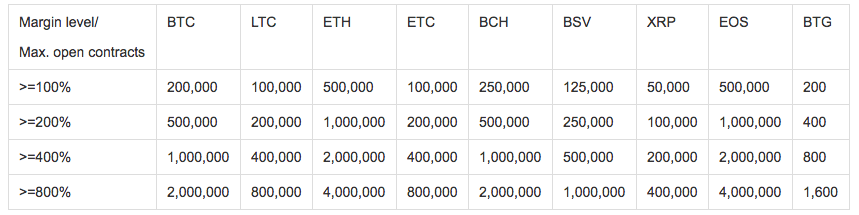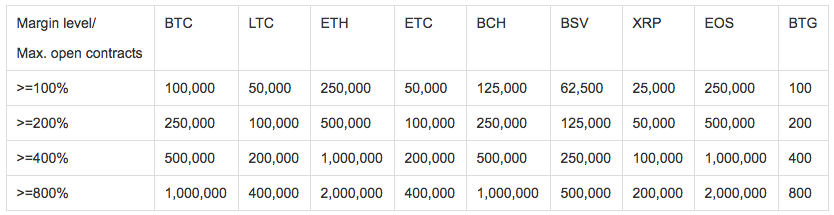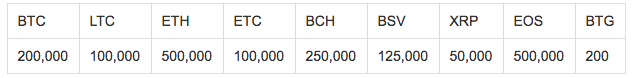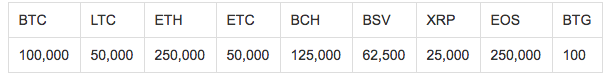# Launching of Position Limit Rules for Futures TradingOKX

To maintain an orderly and stable futures market, and also to prevent any attempts of market manipulation, we are launching the Position Limit Rules tonight. Details are as follows:

Cross-margin Mode: Adopting a scaling margin ratio. Higher margin ratios will be required for opening bigger positions. Therefore, the cost of maliciously manipulating the market will be maximized, thus avoiding such behavior.

For 10x leverage:For 20x leverage:Example

If a user chooses cross-margin mode, 10x leverage, and has a margin level between 100% to 200%, the maximum number of BTC contracts he can open is 200,000, which includes all pending and holding weekly, bi-weekly, and quarterly contracts.

Fixed-margin Mode: Applying maximum limits on opening positions per account. This will prevent the potential market risks triggered by the opening of unusually large positions.

The maximum number of contracts can be opened per account for 10x leverage:The maximum number of contracts can be opened per account for 20x leverage:Example
If a user chooses fixed-margin mode and 10x leverage, the maximum number of weekly BTC contracts he can open is 200,000.

Moreover, to allow our users to have a better understanding of the concept of margin call coefficient, we will also adjust the margin ratio formulae.

Existing Margin Ratio Formulae:

The coefficient of 10x leverage is 10%;
The coefficient of 20x leverage is 20%.

Fixed-margin Margin Ratio = (Margin + UPL)/Position Margin - Adjustment Coefficient
Cross-margin Margin Ratio = Equity Balance/Position Margin - Adjustment Coefficient

If the trader’s margin ratio breaches 0%, a margin call will be triggered

New Margin Ratio Formulae:

Fixed margin Margin Ratio = (Fixed Margin + UPL) * Avg Price of Open Positions / (Contract Face value * Holding Positions)
Cross-margin Margin Ratio = Equity Balance / Position Margin + Withholding Margin of working orders)

If the trader’s margin ratio breaches 10% for 10x leverage, a margin call will be triggered.
If the trader’s margin ratio breaches 20% for 20x leverage, a margin call will be triggered.

The above new formulae are only a conceptual adjustment, and there is no actual change in the conditions of triggering a forced liquidation.

We will continue to make our best efforts to accelerate the optimization of our futures trading platform and also the enhancement of the risk management framework. As always, we appreciate and welcome any thoughts you may have. Should you have any comments or suggestions, please email us at futures@OKX.com.